## Overview

Typically, when developers talk about machine learning (ML), they refer to neural networks (NNs). The great advantage of neural networks is that you do not need to be a domain expert and can quickly get a working solution. The drawbacks of neural networks are that they often require numerous memory and cycles, and that it is difficult to explain how they have reached their conclusion.

The field of machine learning includes technologies other than neural networks. Those other technologies might have been used under a different name, for example statistical machine learning. In this guide, we use the name classical machine learning to refer to the use of those other technologies in the CMSIS-DSP open-source libraries.

The CMSIS-DSP library is a rich collection of DSP functions that Arm has optimized for various Arm Cortex-M processors, for example the Cortex-M4, Cortex-M7, Cortex-M33, Cortex-M35, and the Cortex-M55 processors. The Arm Developer website includes more information and supporting resources for these processors.

CMSIS-DSP is widely used in the industry, and enables optimized C code generation from various third-party tools. Arm has recently added new functions to the CMSIS-DSP library for classical ML, including Support Vector Machine (SVM), naive gaussian Bayes classifier and distances for clustering.

This guide explains how to train the SVM and Bayes classifiers in Python, how to dump the parameters, and how to use the dumped parameters in CMSIS-DSP. It also explains how the distance functions can be used for building clustering algorithms.

These classifiers can be used for anomaly detection, sound classification, and image recognition. They will require the use of smart features, for example the output of a signal processing chain, an understanding of the domain, and will work with fewer classes than neural networks.

The classical ML functions provided in CMSIS-DSP are only available with float32.

### Before you begin

To complete this guide, you should know how to build CMSIS-DSP.

You also need to have the following resources installed:

If you want to display pictures, you should also install matplotlib in Python.

Note: The new classical ML functions are not included by default in Arm Keil MDK or Arm Development Studio projects. To use those functions, you will have to rebuild the library and include them.

The new functions are contained in the following CMSIS-DSP folders:

• SVMFunctions
• BayesFunctions
• DistanceFunctions
• SupportFunctions
• StatisticsFunctions

## What is a Support Vector Machine?

The idea of a Support Vector Machine (SVM) is simple: To separate two clusters of points using a line, as you can see in the following image. The black line is separating cluster B from cluster A: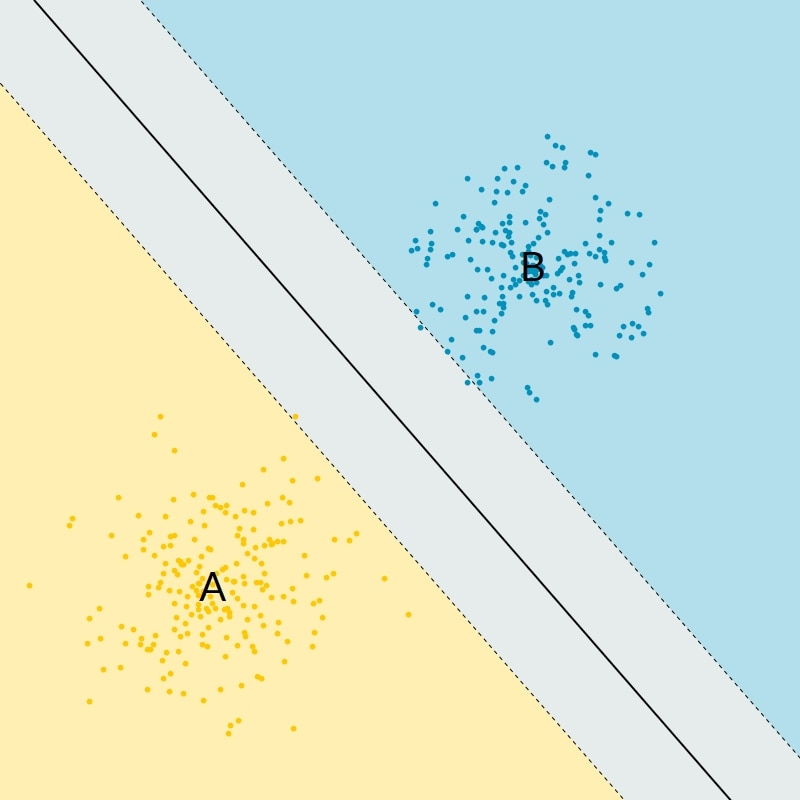Figure 1: Simple linear SVM classifier

The SVM classifier is a binary classifier. There are only two classes. In practice the points are not often points in a plane. Instead the points are feature vectors in a higher-dimensional space and therefore the line is a hyperplane.

Also, there is no reason for the two clusters of points to be separable with a hyperplane, as you can see in the following image:Figure 2: Two clusters of points: Cluster A and Cluster B

Cluster A and B cannot be separated by a plane. To solve this issue, SVM algorithms introduce nonlinear transformations.

In CMSIS-DSP, four kinds of transformations are supported and therefore four kinds of SVM classifiers are available. Those classifiers use vectors, the support vectors, and coefficients, named dual coefficients, which are generated by the training process.

### The linear classifier

The linear prediction uses the following formula:Support vectors xi and dual coefficients are generated during the training. The vector to be classified is y. <x,y> is the scalar product between vectors x and y.

The sign of this expression is used to classify the vector y as belonging to class A or B.

### Polynomial classifier

The polynomial classifier uses the following formula:This formula is more complex than the one for the linear classifier. Several new parameters are generated during the training:

• Gamma
• coef0
• The degree d of the polynomial

The radial basis function classifier uses the following formula:Instead of using a scalar product, Euclidean norm is used. A radial basis function is a function with a value that depends on the distance to a fixed reference point: In this case, the distance between xi and the value to be classified y.

### Sigmoid

The sigmoid classifier uses the following formula:This formula is like the polynomial formula, but instead of computing the power of the expression, tanh is used.

Because the polynomial SVM is the SVM classifier that requires the most parameters, we use the polynomial SVM as an example in this guide. You will learn how to train the polynomial classifier in Python and how to dump the parameters to use the trained classifier in CMSIS-DSP.

## Train an SVM classifier with scikit-learn

In this section of the guide, we focus on how to train an SVM classifier with scikit-learn and how to dump the parameters for use with CMSIS-DSP. The data generation and visualization parts of this activity are beyond the scope of this guide.

The code below can be found in the CMSIS-DSP library:
CMSIS/DSP/Examples/ARM/arm_svm_example/train.py

You can run this example to reproduce the results of this guide, so that you can generate the data, train the classifier and display some pictures.

Let's look at the parts of this script that correspond to the training and dumping of parameters.

The training of the SVM classifier relies on the scikit-learn library. So, we must import svm from the sklearn module.

Training requires some data. The random, numpy, and math Python modules are imported for the data generation part . More modules are required for the graphic visualization. This is described in the train.py file.

The following Python code loads the required modules:

```from sklearn import svm
import random
import numpy as np
import math```

The data is made of two clusters of 100 points. The first cluster is a ball that is centered around the origin. The second cluster has the shape of an annulus around the origin and the previous ball.

This image shows what those clusters of points look like: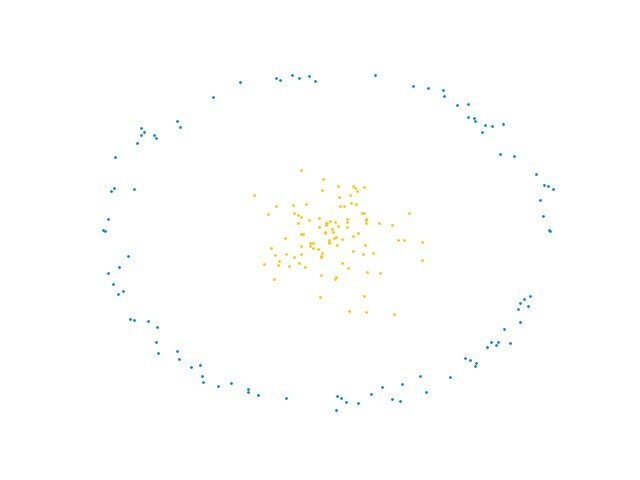Figure 3: Two clusters of points that are not linearly separable

The cluster of points were generated with the following Python code. This code generates the random points and prepares the data for the training of the classifier. The data is an array of points and an array of corresponding classes: X_train and Y_train

The yellow points correspond to class 0 and the blue points correspond to class 1.

```NBVECS = 100
VECDIM = 2

x = ballRadius * np.random.randn(NBVECS, 2)

angle = 2.0 * math.pi * np.random.randn(1, NBVECS)
radius = 3.0 + 0.1 * np.random.randn(1, NBVECS)

xa = np.zeros((NBVECS,2))
xa[:, 0] = radius * np.cos(angle)
xa[:, 1] = radius * np.sin(angle)

X_train = np.concatenate((x, xa))
Y_train = np.concatenate((np.zeros(NBVECS), np.ones(NBVECS)))```

The following two lines create and train a polynomial SVM classifier using the data that we just defined:

`clf = svm.SVC(kernel='poly', gamma='auto', coef0=1.1)clf.fit(X_train, Y_train)`

You can see the result of the training in the following image: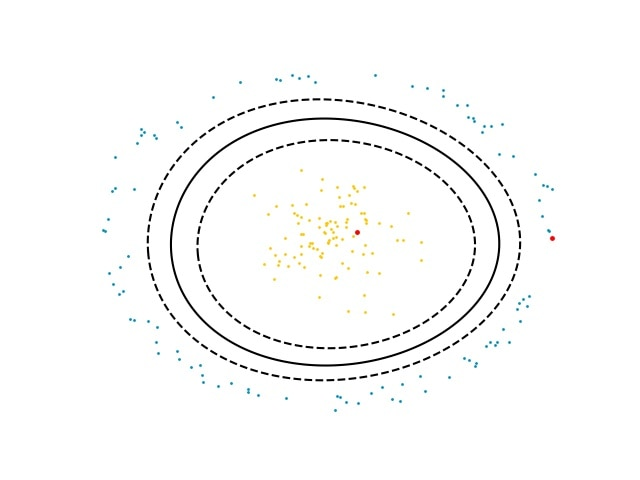Figure 4: Polynomial SVM frontier separating two clusters of points

The solid line represents the separation between the two classes, as the SVM classifier learned.

The larger red points on the image are two test points that are used to check the classifier.

The red point near the center of the image is inside class 0. The red point near the edge of the image corresponds to class 1.

The following code creates the first point inside the center cluster, the class 0, and applies the classifier. The result of predicted1 should be 0:

```test1 = np.array([0.4,0.1])
test1 = test1.reshape(1,-1)

predicted1 = clf.predict(test1)
print(predicted1)```

Now, we would like to use this trained classifier with the CMSIS-DSP. For this, the parameters of the classifier must be dumped.

The CMSIS-DSP polynomial SVM uses the instance structure that is shown in the following code. The parameters of this structure are needed by CMSIS-DSP and must be dumped from the Python script:

```typedef struct
{
uint32_t        nbOfSupportVectors;     /**< Number of support vectors */
uint32_t        vectorDimension;        /**< Dimension of vector space */
float32_t       intercept;              /**< Intercept */
const float32_t *dualCoefficients;      /**< Dual coefficients */
const float32_t *supportVectors;        /**< Support vectors */
const int32_t   *classes;               /**< The two SVM classes */
int32_t         degree;                 /**< Polynomial degree */
float32_t       coef0;                  /**< Polynomial constant */
float32_t       gamma;                  /**< Gamma factor */
} arm_svm_polynomial_instance_f32;```

Other SVM classifiers, for example linear, sigmoid, and rbf, are used in a similar way but require fewer parameters than the polynomial one. This means that, as soon as you know how to dump parameters for the polynomial SVM, you can do the same for other kinds of SVM classifiers.

The following Python script accesses the parameters from the trained SVM classifier and prints the values for use in CMSIS-DSP:

```supportShape = clf.support_vectors_.shape

nbSupportVectors = supportShape
vectorDimensions = supportShape

print("nbSupportVectors = %d" % nbSupportVectors)
print("vectorDimensions = %d" % vectorDimensions)
print("degree = %d" % clf.degree)
print("coef0 = %f" % clf.coef0)
print("gamma = %f" % clf._gamma)

print("intercept = %f" % clf.intercept_)```

Support vectors and dual coefficients are arrays in CMSIS-DSP. They can be printed with the following code:

```dualCoefs = clf.dual_coef_
dualCoefs = dualCoefs.reshape(nbSupportVectors)
supportVectors = clf.support_vectors_
supportVectors = supportVectors.reshape(nbSupportVectors * VECDIM)

print("Dual Coefs")
print(dualCoefs)

print("Support Vectors")
print(supportVectors)```

## Implement your SVM with CMSIS-DSP

Once the parameters of the SVM classifier have been dumped from the Python code, you can use them in your C code with the CMSIS-DSP.

You can find the full code in
CMSIS/DSP/Examples/ARM/arm_svm_example/arm_svm_example_f32.c

This example reproduces the Python prediction by using the same test points. The following code declares the instance variable that used by the SVM classifier and some lengths.

This instance variable will contain all the parameters which have been dumped from Python.

Some of those parameters are arrays so we must specify some sizes, for example, the number of support vectors and their dimensions.

The following code defines the instance variable and some sizes, which will be useful later when creating the arrays.

```arm_svm_polynomial_instance_f32 params;

#define NB_SUPPORT_VECTORS 11
#define VECTOR_DIMENSION 2```

The following code defines 2 arrays. The array of dual coefficients and support vectors is filled with the values coming from Python. The classes 0 and 1 are also defined to ease the comparison with Python:

```const float32_t dualCoefficients[NB_SUPPORT_VECTORS] = {
-0.01628988f, -0.0971605f,
-0.02707579f,  0.0249406f,
0.00223095f,   0.04117345f,
0.0262687f,    0.00800358f,
0.00581823f,   0.02346904f,
0.00862162f};      /**< Dual coefficients */

const float32_t supportVectors[NB_SUPPORT_VECTORS*VECTOR_DIMENSION] = {
1.2510991f,   0.47782799f,
-0.32711859f, -1.49880648f,
-0.08905047f,  1.31907242f,
1.14059333f,  2.63443767f,
-2.62561524f,  1.02120701f,
-1.2361353f,  -2.53145187f,
2.28308122f, -1.58185875f,
2.73955981f,  0.35759327f,
0.56662986f,  2.79702016f,
-2.51380816f,  1.29295364f,
-0.56658669f, -2.81944734f};        /**< Support vectors */

const int32_t   classes = {0,1};```

The following code initializes the instance variable with all the parameters that come from Python, for example, the lengths, the above arrays, and the intercept, degree, coef0 and gamma parameters:

```arm_svm_polynomial_init_f32(¶ms,
NB_SUPPORT_VECTORS,
VECTOR_DIMENSION,
-1.661719f, /* Intercept */
dualCoefficients,
supportVectors,
classes,
3, /* degree */
1.100000f, /* Coef0 */
0.500000f /* Gamma */
);```

Finally, for testing, an input vector is defined and classified using the polynomial SVM predictor.

The following code defines the input vector and applies the classifier:

```in = 0.4f;
in = 0.1f;
arm_svm_polynomial_predict_f32(¶ms,
in,
&result);```

The input vector is a point. This point is defined to be in the center cluster, which corresponds to class 0. This point has the same coordinates as the point which was used in the Python code to test the classifier. So the result of the above code should be the class 0.

An SVM classifier is a binary classifier. If you want to work with more classes, you need to create classifiers for each distinct pair of classes, and use a majority voting on the results to select the final class.

For instance, in the following example from scikit-learn, SVM is used to recognize digits. With ten digits, there are 45 pairs. This means that there are 45 SVM classifiers. Scikit-learn creates them automatically using the strategy one-vs-one: each classed is compared with every other class.

In this case, the extraction of the parameters is more complex because scikit-learn returns matrixes containing parameters for all 45 of the classifiers. In CMSIS-DSP you need 45 instance variables and you extract the values from the matrixes to initialize all those instance variables.

## What is a Bayesian estimator?

An estimator is Bayesian if it uses the Bayes theorem to predict the most likely class of some observed data.

Because the class of data is an unknown parameter and not a random variable, it is not possible to express the probability of that class using the standard concept of probability.

Bayesian probability uses a different notion of probability which quantifies our state of knowledge about the truth of an assertion.

With standard probability, the conditional probability of some random variable X, assuming some value of an unknown parameter theta, cannot be inverted.

You can write the following formula because X is a random variable: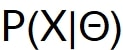But you cannot write the following formula, because theta is not a random variable, but is an unknown parameter: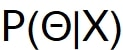Bayesian probability allows you to express the probability that some logical assertions are true.

This means that there is a symmetry in the conditional probability of A assuming B which is expressed with following formula:A and B are both logical assertions. Therefore, the previous formula can be inverted using the Bayes theorem to get the probability of B assuming A.

If A and B are the observed data D and the class of the data C, the Bayes theorem allows you to relate what you know about the data and the classes.

Bayes theorem allows you to compute the most likely class for some observed data, if you know something about how the data depends on the classes.

The probability of a class assuming some observed data is expressed as P(C|D). The probability of the data assuming some class C is expressed as P(D|C).

The Bayes theorem is shown here:For the naive gaussian Bayesian classifier, the data, D, is a vector of samples. We assume that the samples are independent and that they follow a Gaussian distribution.

The parameters of the gaussian for each class will be computed during the training of the Python classifier. For each gaussian, the mean and standard deviation is computed.

Also, if some information about the class is available, and some classes are more or less likely, then this knowledge is encoded in the prior probability P(C).

The Python code also returns a value epsilon, which is needed for numerical accuracy.

## Train your Bayesian estimator with scikit-learn

In this section of the guide, we describe how to train the Bayesian classifier with scikit-learn and how to dump the parameters for use with CMSIS-DSP. The data generation and visualization parts of this activity are beyond the scope of this guide.

The file CMSIS/DSP/Examples/ARM/arm_bayes_example/train.py contains all the code for this example.

You can run this file to reproduce the results of this guide, and to generate the data and train the classifier.

In the example, there are three clusters: A, B, and C. The samples in each cluster are generated using a gaussian distribution.

The following image displays the three clusters of points:Figure 5: Three clusters of points: A, B, and C

The training of the Bayesian classifier is relying on the scikit-learn library. So, we must import GaussianNB from the sklearn.naive_bayes module.

Training requires some data. The random, numpy, and math Python modules are imported for the data generation part of this exercise.

The following Python code loads the required modules:

```from sklearn.naive_bayes import GaussianNB
import random
import numpy as np
import math```

The following code generates three clusters of points:

```# 3 cluster of points are generated
x1 = [1.5, 1] +  ballRadius * np.random.randn(NBVECS,VECDIM)
x2 = [-1.5, 1] + ballRadius * np.random.randn(NBVECS,VECDIM)
x3 = [0, -3] + ballRadius * np.random.randn(NBVECS,VECDIM)```

All the points and their classes are concatenated for the training.

Cluster A is class 0, cluster B is class 1, and cluster C is class 2.

The following code creates the array of inputs by concatenating the three clusters. This code also creates the array of outputs by concatenating the class numbers:

```# All points are concatenated
X_train=np.concatenate((x1,x2,x3))

# The classes are 0,1 and 2.
Y_train=np.concatenate((np.zeros(NBVECS),np.ones(NBVECS),2*np.ones(NBVECS)))```

The following code trains the Gaussian Naïve Bayes classifier on the input arrays that were just created:

```gnb = GaussianNB()
gnb.fit(X_train, Y_train)```

We can check the result by classifying a point in each cluster.

The following code checks that a point in cluster A is recognized as being in cluster A. The class number of cluster A is 0. This means that y_pred should be 0 when this code is executed:

```y_pred = gnb.predict([[1.5,1.0]])
print(y_pred)```

Now, we want to use this trained classifier with the CMSIS-DSP. For this, the parameters of the classifier must be dumped.

The CMSIS-DSP Bayesian classifier uses the instance structure that is shown in the following code. The parameters of this structure are needed by CMSIS-DSP and must be dumped from the Python script:

```typedef struct
{
uint32_t vectorDimension;  /**< Dimension of vector space */
uint32_t numberOfClasses;  /**< Number of different classes  */
const float32_t *theta;          /**< Mean values for the Gaussians */
const float32_t *sigma;          /**< Variances for the Gaussians */
const float32_t *classPriors;    /**< Class prior probabilities */
float32_t epsilon;         /**< Additive value to variances */
} arm_gaussian_naive_bayes_instance_f32;```

The parameters that are required can be dumped with following Python code:

```print("Parameters")
# Gaussian averages
print("Theta = ",list(np.reshape(gnb.theta_,np.size(gnb.theta_))))

# Gaussian variances
print("Sigma = ",list(np.reshape(gnb.sigma_,np.size(gnb.sigma_))))

# Class priors
print("Prior = ",list(np.reshape(gnb.class_prior_,np.size(gnb.class_prior_))))

print("Epsilon = ",gnb.epsilon_)```

## Implement your Bayesian estimator with CMSIS-DSP

Once the parameters of the Bayesian classifier have been dumped from the Python code, you can use them in your C code with the CMSIS-DSP.

You can find the full code in CMSIS/DSP/Examples/ARM/arm_bayes_example/arm_bayes_example_f32.c

This example reproduces the Python prediction by using the same test points.

The following code declares the instance variable that is used by the Bayesian classifier and some lengths.

This instance variable contains all the parameters which have been dumped from Python.

Some of those parameters are arrays. This means that we must specify their sizes, using the number of classes and the vector dimensions:

```arm_gaussian_naive_bayes_instance_f32 S;

#define NB_OF_CLASSES 3
#define VECTOR_DIMENSION 2```

Three arrays of parameters are required:

• Gaussian averages (theta)
• Gaussian variances (sigma)
• The class prior probabilities

The following code defines the content of the arrays. The values are dumped from Python:

```const float32_t theta[NB_OF_CLASSES*VECTOR_DIMENSION] = {
1.4539529436590528f, 0.8722776016801852f,
-1.5267934452462473f, 0.903204577814203f,
-0.15338006360932258f, -2.9997913665803964f
}; /**< Mean values for the Gaussians */

const float32_t sigma[NB_OF_CLASSES*VECTOR_DIMENSION] = {
1.0063470889514925f, 0.9038018246524426f,
1.0224479953244736f, 0.7768764290432544f,
1.1217662403241206f, 1.2303890106020325f
}; /**< Variances for the Gaussians */

const float32_t classPriors[NB_OF_CLASSES] = {
0.3333333333333333f, 0.3333333333333333f, 0.3333333333333333f
}; /**< Class prior probabilities */```

The following code fills the instance variable fields with the arrays, some sizes, and the epsilon value coming from Python.

```S.vectorDimension = VECTOR_DIMENSION;
S.numberOfClasses = NB_OF_CLASSES;
S.theta = theta;
S.sigma = sigma;
S.classPriors = classPriors;
S.epsilon=4.328939296523643e-09;```

Once the data structure is initialized, it is possible to use the classifier. The classifier estimates the probability of each class. However, this classifier is not a good estimator. This means that the values of the probabilities should not be used, except to find the max probability which gives the most likely class for the sample.

The following code tests the classifier on a sample point that is inside cluster A corresponding to class 0.

To find the class, the code looks for the maximum probability, which is giving the most likely class.

```in = 1.5f;
in = 1.0f;

arm_gaussian_naive_bayes_predict_f32(&S, in, result);

arm_max_f32(result,
NB_OF_CLASSES,
&maxProba,
&index);

printf("Class = %d\n",index);```

## What is clustering?

Clustering is an attempt to partition a set of points into different clusters of similar points. To be able to do this, we need a method to measure how close or similar the points are. Many clustering algorithms rely on a distance function for that purpose.

The following figure shows the result of a clustering algorithm using three different distance functions, and trying to identify five different clusters from uniformly distributed points.Figure 6: Clusters that depend on the choice of the distance function

CMSIS-DSP does not provide any clustering algorithm. Instead CMSIS-DSP provides the distance functions which can be used to build clustering algorithms.

Building a clustering classifier using distances requires a way to quickly find the distance between one point and other points. Strategies for this are beyond the scope of the CMSIS-DSP library.

## Use CMSIS-DSP distance functions

CMSIS-DSP provides most of the distance functions which are generally used In clustering algorithms. It includes distance functions for float but also for booleans.

All distance functions in the CMSIS-DSP have a similar API. Let's use the Manhattan distance, also known as the city block distance, as an example.

The API of the city block distance is described in the following code:

```float32_t arm_cityblock_distance_f32(const float32_t *pA,
const float32_t *pB,
uint32_t blockSize);```

This function computes the distance between two vectors, pA and pB, of the dimension blockSize.

The folder CMSIS/DSP/DistanceFunctions also contains functions which are not really distances from a mathematical point of view. For instance, the cosine distance is a measure of similarity.

## Miscellaneous new CMSIS-DSP functions

The functions that are listed in this section of the guide are useful if you are building more complex Classical-ML algorithms.

CMSIS-DSP introduces two new functions that are useful if you are computing weighted sums of points or scalars.

arm_ barycenter_f32
Is a utility function that computes the barycenter of some weighted points.
arm_weighted_sum_f32
Works with scalars and computes the weighted sum of those scalars.

The following code describes the API of those functions:
```void arm_barycenter_f32(const float32_t *in,
const float32_t *weights,
float32_t *out,
uint32_t nbVectors,
uint32_t vecDim);

float32_t arm_weighted_sum_f32(const float32_t *in,
const float32_t *weigths,
uint32_t blockSize);```
CMSIS-DSP introduces two new functions that are related to entropy.
arm_entropy_f32
Computes the entropy of a probability distribution pSrcA.
arm_kullback_leibler_f32
Computes the Kullback Leibler divergence between two probability distributions.

The following code describes the API of those functions:
```float32_t arm_entropy_f32(const float32_t * pSrcA,
uint32_t blockSize);

float32_t arm_kullback_leibler_f32(const float32_t * pSrcA,
const float32_t * pSrcB,
uint32_t blockSize);```
When working with Gaussian probability, rounding issues can become a problem. This is because the dynamic of the values can be large. Instead, you can work with the log of the values.
arm_logsumexp_f32
Computes the sum of probabilities represented by their log. This sum is computed considering accuracy issues.
arm_logsumexp_dot_prod_f32
Computes the dot product when the values are represented by their log.

When working with conditional probabilities, represented as tables, you often need to compute dot products between the row and the columns of those matrixes. If the probabilities are represented by their log values you'll need to use a function like arm_logsumexp_dot_prod_f32.

The following code describes the API of those functions:
```float32_t arm_logsumexp_dot_prod_f32(const float32_t * pSrcA,
const float32_t * pSrcB,
uint32_t blockSize,
float32_t *pTmpBuffer);

float32_t arm_logsumexp_f32(const float32_t *in, uint32_t blockSize);```

## Related information

Here are some resources related to material in this guide:

## Next steps

This guide has explained how to implement some classical machine learning classifiers using the CMSIS-DSP. The guide has shown how to train the classifiers in Python, how to dump the parameters, and how to use the dumped parameters in CMSIS-DSP.

If you want to explore these concepts further, Kaggle has some useful applications of those classifiers.

Thank you for reading our guide on implementing classical ML classifiers with CMSIS-DSP. We look forward to seeing what you can create with CMSIS-DSP.

If you have any questions when using CMSIS-DSP, create a GitHub issue.